# KR100558481B1 - Delta-sigma modulator that can reduce quantization noise - Google Patents

## Info

Publication number
KR100558481B1
KR100558481B1 KR20030000354A KR20030000354A KR100558481B1 KR 100558481 B1 KR100558481 B1 KR 100558481B1 KR 20030000354 A KR20030000354 A KR 20030000354A KR 20030000354 A KR20030000354 A KR 20030000354A KR 100558481 B1 KR100558481 B1 KR 100558481B1
Authority
KR
South Korea
Prior art keywords
output signal
signal
bit quantizer
digital
sigma modulator
Prior art date
Application number
KR20030000354A
Other languages
Korean (ko)
Other versions
KR20040062823A (en
Inventor
이용희
Original Assignee
삼성전자주식회사
Priority date (The priority date is an assumption and is not a legal conclusion. Google has not performed a legal analysis and makes no representation as to the accuracy of the date listed.)
Filing date
Publication date
Application filed by 삼성전자주식회사 filed Critical 삼성전자주식회사
Priority to KR20030000354A priority Critical patent/KR100558481B1/en
Publication of KR20040062823A publication Critical patent/KR20040062823A/en
Application granted granted Critical
Publication of KR100558481B1 publication Critical patent/KR100558481B1/en

## Images

•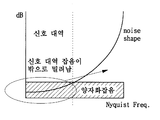•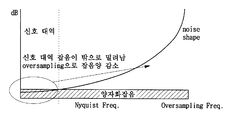•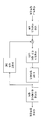•••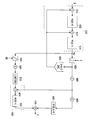•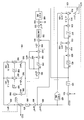•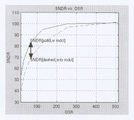•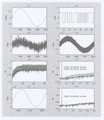•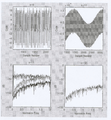## Classifications

• HELECTRICITY
• H03BASIC ELECTRONIC CIRCUITRY
• H03MCODING; DECODING; CODE CONVERSION IN GENERAL
• H03M3/00Conversion of analogue values to or from differential modulation
• H03M3/30Delta-sigma modulation
• H03M3/458Analogue/digital converters using delta-sigma modulation as an intermediate step
• H03M3/46Analogue/digital converters using delta-sigma modulation as an intermediate step using a combination of at least one delta-sigma modulator in series with at least one analogue/digital converter of a different type

## Abstract

The present invention relates to a delta sigma modulator, comprising a 1-bit quantizer, a multi-bit quantizer and an error correction circuit, and correcting an error using a digital output signal of a multi-bit quantizer and a digital output signal of a 1-bit quantizer. It is characterized by reducing the quantization noise generated when converting the signal into a digital output signal.
According to the delta sigma modulator according to the present invention, the quantization noise can be reduced by correcting an error having a great influence on characteristics by using the digital output signal of the multi-bit quantizer and the digital output signal of the 1-bit quantizer. In addition, according to the delta sigma modulator according to the present invention, it is possible to eliminate the DC offset caused by the multi-bit quantizer.

## Description

Delta Sigma MODULATOR THAT CAN REDUCE QUANTIZATION NOISE to Reduce Quantization Noise

FIG. 1 (a) is a diagram illustrating a method of reducing noise in a signal band using a noise shaping technique.

FIG. 1B is a diagram illustrating a method of reducing noise in a signal band using an oversampling technique and a noise shaping technique.

2 is a block diagram of a conventional A / D converter implemented using a delta sigma modulator.

3 is a diagram illustrating a conventional delta sigma modulator using a 1-bit quantizer and a multi-bit quantizer, and implemented by correcting quantization noise in an analog manner.

4 is a diagram illustrating a delta sigma modulator according to a first embodiment of the present invention implemented using a 1-bit quantizer and a multi-bit quantizer.

5 is a diagram illustrating a delta sigma modulator according to a second embodiment of the present invention implemented using a 1-bit quantizer and a multi-bit quantizer.

FIG. 6 is a diagram illustrating a detailed transfer function of the delta sigma modulator illustrated in FIG. 5.

7 is a diagram illustrating a simulation result of signal-to-noise ratio according to oversampling ratio for a delta sigma modulator according to the present invention and a delta sigma modulator without conventional quantization noise correction.

8 and 9 are diagrams illustrating a matlab system simulation result for a delta sigma modulator according to the present invention and a delta sigma modulator without conventional quantization noise correction.

<Description of the symbols for the main parts of the drawings>

410: input integration circuit series 411, 413, 451, 470: subtractor

412, 414: Integrator 420: 1-bit quantizer

430: 1-bit D / A converter 440: Multi-bit quantizer

450: error correction circuit 460: delay element

480, 490, 500: gain factor 510: limiter

The present invention relates to a delta sigma modulator, and more particularly to a delta sigma modulator capable of reducing quantization noise by correcting errors in the digital domain.

In general, the delta sigma A / D converter or delta sigma D / S consisting of a delta-sigma modulator and a digital filter at the input / output or transmit end of certain applications that operate at low frequencies and require high resolution. Signal conversion using A converter. Delta sigma modulators use oversampling and noise shaping. FIG. 1 (a) shows a method for reducing noise in a signal band using a noise shaping technique, and FIG. 1 (b) shows a method for reducing noise in a signal band using an oversampling technique and a noise shaping technique. have. Noise shaping technology pushes the quantization noise that occurs during signal conversion into an unused signal band, the amount of which depends on the order of the delta sigma modulator. Oversampling is a technique in which the quantization noise is increased in all bands to reduce the quantization noise in the actual signal band. In addition, the delta sigma modulator acts as a highpass filter and keeps the signal band high through oversampling, so that the noise ratio of the signal band to be converted in a small order is relatively small to improve the signal-to-noise ratio within the signal band. Can be. In other words, the delta sigma modulation method is a method that can obtain a high resolution by reducing the noise in the signal band using a noise shaping technique and an oversampling technique.

2 is a block diagram of a conventional A / D converter implemented using a delta sigma modulator. The characteristic of the A / D converter of FIG. 2 is determined by the order L of the analog loop filter and the number N of bits of the quantizer when the oversampling ratio (OSR) is constant. In general, an A / D converter implemented using a delta sigma modulator uses a 1-bit quantizer to avoid the nonlinear characteristics of the quantizer. Thus, an A / D converter implemented using a delta sigma modulator is also called a 1-bit A / D converter. In general, an inverse relationship is established between the order L of the modulator and the OSR to obtain the proper noise ratio characteristics required for the application. For example, in applications in the voice band (4 kHz, Fs = 8 kHz), L = 2, OSR = 256, N = 1 is appropriate, and audio band (20 kHz, Fs = 44.1 kHz), L = 4, OSR = 64, and N = 1 are appropriate. Where Fs is the sampling frequency. The deterioration of characteristics caused by the small L value can be canceled with each other by raising the OSR.

However, since the oversampling technique operates at a frequency higher than the Nyquist frequency by OSR, there is a problem in implementing a circuit in an application having a high signal band. If the OSR is 256 in an application where the Nyquist frequency is 2 MHz, the operating frequency becomes 512 MHz, which is a problem.In order to lower the OSR, the order of the modulator (L) is increased, so the noise, power consumption, And various problems in terms of product cost. In particular, in the case of an analog modulator, since most circuits are composed of analog circuits which are sensitive to noise, they are difficult to implement under the influence of noise.

In order to solve this problem, various methods have recently been proposed to exhibit proper characteristics even for low OSR and low order of modulator. An important method of these proposals is to use a multi-stage noise shaping (MASH) method that adds, filters, and removes quantization noise at each stage, and a dual quantizer of 1 and N bits. For example, the analog quantization noise generated from the bit quantizer is removed and the output is implemented through digital noise shaping. A method of removing quantization noise using digital correction after using a dual quantizer of 1 bit and N bits is disclosed in US Pat. No. 6,300,890 and shown in FIG. The conventional delta sigma modulator shown in FIG. 3 can quantize an analog input signal by a feedback loop comprising a 1 bit quantizer, and perform quantization noise correction using a loop comprising a multiple bit quantizer 11. This can reduce the quantization noise. In the delta sigma modulator of FIG. 3, the quantized analog output signal of the 1 bit D / A converter 4 is subtracted from the analog output signal of the final stage integrator 8 to calculate the quantization error. This eliminates the quantization noise from the A converter. However, since the signal subtracted by the subtractor 10 is an analog signal and the loop of the signal for removing quantization noise is an analog circuit, it may be affected by noise.

In order to solve the problems as described above, the delta sigma modulator according to the present invention includes a 1-bit quantizer, a multi-bit quantizer and an error correction circuit, and the digital output signal of the multi-bit quantizer and the digital output signal of the 1-bit quantizer By correcting the error in the digital domain, the quantization noise can be reduced, and the loop of the correction circuit is performed in the digital domain, thereby minimizing the influence on the noise.

It is an object of the present invention to provide a delta sigma modulator that reduces quantization noise and minimizes the effects of noise.

It is another object of the present invention to provide a delta sigma modulator that can eliminate the DC offset that may be caused by a multi-bit quantizer.

The delta sigma modulator according to the present invention includes a 1-bit quantizer, a multi-bit quantizer, and an error correction circuit, and corrects an error using a digital output signal of the multi-bit quantizer and a digital output signal of the 1-bit quantizer. When the signal is converted into a digital output signal, the quantization noise is corrected and reduced.

The delta sigma modulator according to the present invention quantizes an input terminal to which an analog input signal is input, an output terminal to which a digital output signal is output, quantizes an analog signal located between the input terminal and the output terminal, and outputs a quantized digital signal. One or more stages including a one bit quantizer for converting the quantized digital signal into a quantized analog signal, an integrator for integrating a first subtractor and an output of the first subtractor Wherein the first subtractor of the first stage subtracts the quantized analog output signal of the 1-bit D / A converter from the analog input signal of the input terminal and the last stage of integrator outputs its output to the 1-bit quantizer Quantize the output signal of the input integration circuit series, A multi-bit quantizer for outputting a magnetized digital signal, an error correction circuit for receiving a digital signal of the multi-bit quantizer and a digital signal of the 1-bit quantizer and correcting an error, and a quantized digital of the 1-bit quantizer And a second subtractor for subtracting the output signal of the error correction circuit from the output signal of the delay element and outputting the result.

The error correction circuit may further include: a third subtractor for subtracting the digital signal of the multi-bit quantizer from the digital signal of the 1-bit quantizer to obtain a correlation between quantization noise generated in the 1-bit quantizer and noise of the multi-bit quantizer; And a differentiator for noise shaping to minimize the amount of noise in a desired signal band by differentiating the output signal of the third subtractor to implement a function of a high pass filter with respect to the amount of noise generated in the bit quantizer. .

The delta sigma modulator further includes a limiter between the multi-bit quantizer and the error correction circuit to remove a DC offset that may be generated by using the multi-bit quantizer.

Hereinafter, a delta sigma modulator according to the present invention will be described with reference to the accompanying drawings.

4 is a diagram illustrating a delta sigma modulator according to a first embodiment of the present invention implemented using a 1-bit quantizer and a multi-bit quantizer.

The delta sigma modulator of FIG. 4 quantizes the quantized and quantized analog signal which is located between the input terminal to which the analog input signal X is input, the output terminal to which the digital output signal Y is output, and between the input terminal and the output terminal. A one bit quantizer 420 for outputting the signal, a one bit D / A converter 430 for converting the quantized digital signal into a quantized analog signal, one or more stages including a subtractor and an integrator Input integration circuit series 410, input integration circuit which subtracts and integrates the quantized analog output signal of the 1-bit D / A converter 430 from the input signal X and outputs the output to the 1-bit quantizer 420. The digital output signal of the multi-bit quantizer 440 and the multi-bit quantizer 440 and the one-bit quantum for quantizing the output signal of the series 410 and outputting the quantized digital signal. An error correction circuit 450 that receives the digital output signal of the device 420 and corrects an error, a delay element 460 for delaying the quantized digital signal of the 1-bit quantizer 420, and a delay element 460 And an subtractor 470 for subtracting the output signal of the error correction circuit 450 and outputting the subtraction result.

The input integration circuit series 410 includes a subtractor 411 for subtracting the analog output signal of the 1-bit D / A converter 430 from the analog input signal, an integrator 412 for integrating the output signal of the subtractor 411, and an integrator ( A subtractor 413 for subtracting the analog output signal of the 1-bit D / A converter 430 from the output signal of 412 and an integrator 414 for integrating the output signal of the subtractor 413.

The error correction circuit 450 subtracts the digital output signal of the multi-bit quantizer 440 from the digital output signal of the 1-bit quantizer 420 to compare the quantization noise generated by the 1-bit quantizer with the noise of the multi-bit quantizer. Differential subtractor 451 and the output signal of subtractor 451 to differentiate the noise generated by the bit quantizer to implement the function of the high pass filter to minimize the amount of noise in the desired signal band ( 452).

Hereinafter, the operation of the delta sigma modulator according to the first embodiment of the present invention will be described with reference to FIG. 4.

In FIG. 4, the input integrating circuit series 410 is composed of one or more steps consisting of a subtractor and an integrator, and FIG. 4 illustrates a second case consisting of two steps. The analog input signal X is quantized by the 1-bit quantizer 420 and converted into a digital signal. A feedback loop consisting of a 1-bit quantizer 420, a 1-bit D / A converter 430, subtractors 411, 413, and integrators 412, 414 allows the noise to be shaped out of the desired signal band and into the desired signal. Relatively less noise and the analog input signal continue to quantize. Since the quantization noise E may be mixed in the quantization process, the output signal of the 1-bit quantizer 420 becomes a signal including the quantization noise E. The multi-bit quantizer 440 receives an output signal of the input integrating circuit series 410, that is, an input signal Vm of the 1-bit quantizer 420 and outputs a quantized digital signal. Like the 1-bit quantizer 420, the multi-bit quantizer 440 may mix quantization noise (EE) during the quantization process, so that the output signal of the multi-bit quantizer 440 is a signal including the quantization noise (E). Becomes The subtractor 451 of the error correction circuit 450 subtracts the output signal of the multi-bit quantizer 440 from the output signal of the 1-bit quantizer 420, and the derivative 452 differentiates the result. The delay element 460 receives the output signal of the 1-bit quantizer 420 and delays a predetermined time. The subtractor 470 subtracts the output signal of the error correction circuit 450 from the output signal of the delay element 460 to generate the output signal Y at the output terminal. In this process, the subtractor 451 obtains a correlation between the quantization noise generated in each bit quantizer, and the differentiator 452 implements a high pass filter function for the amount of noise generated in each bit quantizer. Functions as noise shaping to minimize volume. As a result, the relatively large 1-bit quantizer noise is eliminated at the final output stage, and the noise of the relatively small multi-bit quantizer is very small through noise shaping by the differentiator, thereby improving the characteristics of the signal-to-noise ratio. .

FIG. 5 is a diagram illustrating a delta sigma modulator according to a second embodiment of the present invention implemented using a 1-bit quantizer and a multi-bit quantizer. The sigma modulator according to the first embodiment of the present invention shown in FIG. A predetermined gain element and limiter for correcting the difference in gain of each step are further provided.

The delta sigma modulator of FIG. 5 quantizes an input signal to which an analog input signal X is input, an output terminal to which a digital output signal Y is output, an analog signal located between an input terminal and an output terminal, and quantizes the quantized digital signal. A one-bit quantizer 420 for outputting the digital signal, a one-bit D / A converter 430 for converting the quantized digital signal into a quantized analog signal, one or more stages consisting of a subtractor and an integrator and Input integration circuit series 410, 1-bit quantizer which subtracts and integrates the quantized analog output signal of 1-bit D / A converter 430 from signal X and outputs the output to 1-bit quantizer 420. A gain element 480 for giving a predetermined gain to the digital output signal of 420, for quantizing the output signal of the input integration circuit series 410, and for outputting a quantized digital signal; The gain element 500 for giving a predetermined gain to the digital output signal of the bit quantizer 440, the multi-bit quantizer 440, the limiter 510 and the limiter 510 for stabilizing the output signal of the gain element 500. An error correction circuit 450 for receiving an output signal of the gain element 480 and an output signal of the gain element 480 to correct an error, a gain element 490 for giving a predetermined gain to the output signal of the gain element 480, and a gain. A delay element 460 for delaying the output signal of the element 490 and a subtractor 470 for subtracting the output signal of the error correction circuit 450 from the output signal of the delay element 460 and outputting a subtraction result. Equipped.

The input integration circuit series 410 includes a subtractor 411 for subtracting the analog output signal of the 1-bit D / A converter 430 from the analog input signal, an integrator 412 for integrating the output signal of the subtractor 411, and an integrator ( A subtractor 413 for subtracting the analog output signal of the 1-bit D / A converter 430 from the output signal of 412 and an integrator 414 for integrating the output signal of the subtractor 413.

The error correction circuit 450 includes a subtractor 451 for subtracting the output signal of the limiter 510 from the output signal of the gain element 480, and a differentiator 452 for differentiating the output signal of the subtractor 451.

The delta sigma modulator according to the second embodiment of the present invention shown in Fig. 5 is designed to remove a DC offset that may be generated by using a multi-bit quantizer and a predetermined gain element for correcting each other's gain difference. Except that it further includes a limiter for limiting the output of the bit quantizer to a constant level, the description thereof is omitted here because it is the same as the delta sigma modulator according to the first embodiment of the present invention shown in FIG.

FIG. 6 is a diagram illustrating in detail the transfer function of the second delta sigma modulator illustrated in FIG. 5.

In FIG. 6, reference numeral 410 denotes an input integration circuit series 410, reference numeral 420 denotes a 1-bit quantizer, reference numeral 430 denotes a 1-bit D / A converter, reference numeral 440 denotes a multi-bit quantizer, and reference numeral 450 denotes An error correction circuit, reference numeral 460 denotes a delay circuit, reference numeral 470 denotes a subtractor, reference numerals 480, 490, and 500 denote gain elements, and 510 denotes a limiter. The same number as in was used. Figures 6, a1 = 1, a2 = 1, a3 = 1, a4 = 0.75, a5 = -1.5, a6 = 1, b1 = 1, b4 = 1, b5 = -2, b6 = 1, c1 = 0.5, c2 = 0.5, g1 = 2 3 , g2 = 2 10 ) represents the coefficient value of the transfer function used in the embodiment of the present invention.

Y1 (z), the sum of the signal transfer function and the noise transfer function, is expressed by Equation 1.

The input signal of the subtractor 451 is g1 (X2 + E)-g1 (X2 + EE) = g1 (E-EE). The signal passing through the multiple bit quantizer 440 has a relatively small quantization noise (EE) compared to the one bit quantizer. When calculated using the gain shown in FIG. 6, the value of X3 is equal to Equation 2.

Since noise E exists in the feedback loop of the delta sigma modulator of FIG. 6, the output Y (z) = f (X (z), E (z), EE (z)). If we remove E inside this function, the output of the delta sigma modulator is Y (z) = f (X (z), EE (z)). EE (z) is an error of the multi-bit quantizer 440 with a relatively small amount of error. In this way, the sigma modulator shown in FIG. 6 exhibits excellent noise characteristics because noise E, which may have the greatest influence on the noise characteristics, can be removed to remove the effects of errors in the feedback loop of the sigma modulator.

In order to remove the quantization noise E of the delta sigma modulator, the output signal Y (z) should be expressed by Equation 3 below.

Using equations (1) and (2), the output signal Y (z) is obtained as shown in equation (4).

It can be seen from Equation 4 that the quantization noise E is canceled out and only the quantization noise EE of the multi-bit quantizer remains. In addition, it can be seen from Equation 4 that the delta sigma modulator required includes a second-order highpass filter having a delay time. The highpass filter is characterized by noise shaping, which pushes the noise (EE (z)) out of the desired band and has a small amount of noise in the desired signal band.

The delta sigma modulator of the present invention as shown in Fig. 6 eliminates the loss of the original signal, removes relatively large quantization noise E and noise shapings the relatively small quantization noise EE, thereby reducing the noise within the desired signal band. It can have excellent signal-to-noise ratio characteristics. In addition, the sigma delta sigma modulator of the present invention may have excellent characteristics by performing noise cancellation in the digital domain even at a low OSR. In FIG. 6, the gains g1 and g2 are used to calculate the signal in bits when the analog signal is transferred to the digital signal, and thus only affects the magnitude of the signal. Therefore, for the signal-to-noise ratio characteristic of M bits or more, the product of g1 and g2 must be a power of (M-1) of at least 2. If you want more than 14 bits, g1 = 2 3 and g2 = 2 10 . After the quantization, the operation is performed in two's complement form in order to perform the operation in the error correction circuit. If the signal is normal when the M bit signal is to be converted, it is + (2 M- 1-1) to-(2 M-1 ). However, since the sum does not become zero when the operation is performed, the DC value appears at the output terminal of the multi-bit quantizer 440. To prevent this, limit the output of the multi-bit quantizer 440 to + (2 M- 1-1) to-(2 M- 1-1) to make the sum zero. This function is performed by limiter 510 which limits the output of the multi-bit quantizer to a constant level.

In the above, a simple second sigma delta modulator has been described as an example. In general, data conversion is performed using a higher-order modulator to obtain excellent characteristics. In this case, a proportional relationship is established between the OSR and the characteristics of the modulator. Therefore, a good OSR should be maintained because low OSR does not produce good characteristics. Using the circuit structure proposed in the present invention, it is possible to remove a relatively large quantization noise even in a higher-order modulator to improve the loss of characteristics caused by the quantization noise. Accordingly, the delta sigma modulator according to the present invention can be applied to a general A / D converter or a D / A converter.

7 is a diagram illustrating a simulation result of a signal-to-noise ratio according to an oversampling ratio for a delta sigma modulator according to the present invention and a conventional delta sigma modulator. In FIG. 7, a solid line represents a simulation result for the second delta sigma modulator implemented using the circuit structure of the present invention, and a dotted line represents a simulation result for a conventional delta sigma modulator. As can be seen in FIG. 7, when the OSR (Over-Sampling Ratio) is appropriate for the order of the modulator, that is, when the OSR is 256 in the example of FIG. 7, the modulator of the present invention and the conventional modulator have almost no difference in characteristics. If it is higher than this value, it will be saturated. However, when the OSR is low, for example, when the OSR is 64 in FIG. 7, it can be seen that the modulator of the present invention and the conventional modulator have a characteristic difference of 15 dB or more. That is, in the condition of having the same OSR in the same order modulator, i.e., in the state of exhibiting sufficient modulator characteristics other than the saturation condition, the modulator of the present invention can improve the characteristic of 2.5 bits or more as compared to the conventional modulator. This is a characteristic improvement similar to that obtained by doubling the OSR to 128. In other words, the modulator of the present invention exhibits the effect of raising the OSR by twice as much as the conventional modulator for the same order under the above conditions.

8 and 9 are diagrams illustrating a matlab system simulation result for a delta sigma modulator according to the present invention and a conventional delta sigma modulator. 8 and 9 show simulation results for the case where the order of the modulator is 2 and OSR is 64. FIG.

In FIG. 8, (s-1) represents an input signal (-0.707 to +0.707), (s-2) represents an output signal of a modulator according to the conventional scheme, and (s-3) represents a modulator of the present invention. Denotes the output signal (4 bit, -7 to +7) of the limiter passing through the multi-bit quantizer, (s-4) denotes the output signal (Y (z)) of the modulator of the present invention, and (s-5 ) Shows the result of FFT the simulation results for the delta sigma modulator according to the present invention and the conventional delta sigma modulator, and (s-6) shows the result of expanding the result of (s-5) only to the signal bandwidth (0.5). (S-7) shows the result of downsampling the output by inserting the output of the delta sigma modulator according to the present invention into a fourth order digital sync filter, and (s-8) The result of FFT of the result of a digital sync filter is shown.

In (s-5), the upper part shows the FFT result for the delta sigma modulator of the present invention, and the lower part shows the FFT result for the conventional delta sigma modulator. As can be seen from (s-6), as a result of expanding the result of (s-5) only to the signal bandwidth (0.5), it can be seen that the signal-to-noise ratio characteristic is improved by 15 dB (2.5 bit).

In FIG. 9, (s-9) shows a result obtained by calculating an error value between the output signal of the 1-bit quantizer and the output signal of the multi-bit quantizer of the delta sigma modulator of the present invention. The digital error correction of the delta sigma modulator of the present invention is shown (Y2 (z)), (s-11) represents the result of FFT for (s-9) and (s-10), (s -12) shows a waveform of the result of the digital sync filter (result of s-8 in FIG. 8).

In (s-11), the top waveform shows the result of FFT the waveform of (s-9), the middle waveform shows the result of FFT the waveform of (s-10), and the bottom waveform shows the delta of the present invention. The waveform of the final output signal of the sigma modulator is shown. The top waveform is a waveform with no noise shaping, and the middle waveform is a waveform with noise shaping. Quantization noise (E), which greatly affects the characteristics of the delta sigma modulator, is removed by error correction and the remaining quantization noise (EE) is removed by highpass filtering in the differentiator.

Although described above with reference to a preferred embodiment of the present invention, those skilled in the art will be variously modified and changed within the scope of the invention without departing from the spirit and scope of the invention described in the claims below I can understand that you can.

As described above, the delta sigma modulator according to the present invention comprises a 1-bit quantizer, a multi-bit quantizer and an error correction circuit, and uses a digital output signal of the multi-bit quantizer and a digital output signal of the 1-bit quantizer. Correcting errors in the digital domain can reduce quantization noise and eliminate DC offsets that can be caused by multi-bit quantizers. In addition, the sigma delta sigma modulator according to the present invention can achieve an improved signal-to-noise ratio, which is difficult to achieve high OSR due to the wide bandwidth of the signal and high-speed operation. Can be used to obtain good characteristics.

## Claims (10)

1. delete
2. An input terminal to which an analog input signal is input;
An output terminal for outputting a digital output signal;
A 1-bit quantizer for quantizing the analog signal located between the input terminal and the output terminal and outputting a quantized digital signal;
A 1-bit D / A converter converting the quantized digital signal into a quantized analog signal;
And one or more stages including an integrator for integrating a first subtractor and an output of the first subtractor, wherein the first subtractor in the first stage comprises the one bit D / A in the analog input signal of the input terminal. An input integrator circuit series for subtracting the quantized analog output signal of the converter and outputting the output to the 1-bit quantizer;
A multi-bit quantizer for quantizing the output signal of the input integration circuit series and outputting a quantized digital signal;
An error correction circuit that receives an error in the digital output signal of the multi-bit quantizer and the digital output signal of the 1-bit quantizer and corrects an error;
A delay element for delaying the quantized digital output signal of the one bit quantizer; And
And a second subtractor for subtracting the output signal of the error correction circuit from the output signal of the delay element and outputting the result.
3. The method of claim 2, wherein the error correction circuit is
A third subtractor for subtracting the digital output signal of the multi-bit quantizer from the digital output signal of the 1-bit quantizer; And
And a differentiator for differentiating the output signal of the third subtractor.
4. The method of claim 2, wherein the delta sigma modulator
And a limiter for limiting output to remove DC offset between the multi-bit quantizer and the error correction circuit.
5. The method of claim 2, wherein the delta sigma modulator
A sigma delta sigma modulator for converting analog signals into digital signals.
6. An input terminal to which an analog input signal is input;
An output terminal for outputting a digital output signal;
A 1-bit quantizer for quantizing the analog signal located between the input terminal and the output terminal and outputting a quantized first digital signal;
A 1-bit D / A converter converting the first quantized digital signal into a quantized analog signal;
And one or more stages including an integrator for integrating a first subtractor and an output of the first subtractor, wherein the first subtractor in the first stage comprises the one bit D / A in the analog input signal of the input terminal. An input integrator circuit series for subtracting the quantized analog output signal of the converter and outputting the output to the 1-bit quantizer;
A first gain element for giving a predetermined gain to the first digital signal of the 1-bit quantizer;
A multi-bit quantizer for quantizing the output signal of the input integration circuit series and outputting a quantized second digital signal;
A second gain element for giving a predetermined gain to a second digital signal of the multi-bit quantizer;
An error correction circuit for receiving an output signal of the second gain element and an output signal of the first gain element to correct an error;
A third gain element for giving a predetermined gain to the output signal of the first gain element;
A delay element for delaying the output signal of the third gain element; And
And a second subtractor for subtracting the output signal of the error correction circuit from the output signal of the delay element and outputting the result.
7. The method of claim 6, wherein the error correction circuit
A third subtractor which subtracts the output signal of the second gain element from the output signal of the first gain element; And
And a differentiator for differentiating the output signal of the third subtractor.
8. 7. The apparatus of claim 6, wherein the delta sigma modulator
A delta sigma modulator further comprising a limiter between the second gain element and the error correction circuit.
9. 7. The apparatus of claim 6, wherein the delta sigma modulator
And the first gain element and the second gain element have the same value.
10. 10. The apparatus of any one of claims 6 to 9, wherein the delta sigma modulator
A sigma delta sigma modulator for converting analog signals into digital signals.
KR20030000354A 2003-01-03 2003-01-03 Delta-sigma modulator that can reduce quantization noise KR100558481B1 (en)

## Priority Applications (1)

Application Number Priority Date Filing Date Title
KR20030000354A KR100558481B1 (en) 2003-01-03 2003-01-03 Delta-sigma modulator that can reduce quantization noise

## Applications Claiming Priority (3)

Application Number Priority Date Filing Date Title
KR20030000354A KR100558481B1 (en) 2003-01-03 2003-01-03 Delta-sigma modulator that can reduce quantization noise
US10/723,159 US6922161B2 (en) 2003-01-03 2003-11-26 Delta-Sigma modulator for reducing quantization noise and oversampling ratio (OSR)
JP2003435973A JP3895325B2 (en) 2003-01-03 2003-12-26 Delta-sigma modulator

## Publications (2)

Publication Number Publication Date
KR20040062823A KR20040062823A (en) 2004-07-09
KR100558481B1 true KR100558481B1 (en) 2006-03-07

# Family

## Family Applications (1)

Application Number Title Priority Date Filing Date
KR20030000354A KR100558481B1 (en) 2003-01-03 2003-01-03 Delta-sigma modulator that can reduce quantization noise

## Country Status (3)

US (1) US6922161B2 (en)
JP (1) JP3895325B2 (en)
KR (1) KR100558481B1 (en)

## Families Citing this family (22)

* Cited by examiner, † Cited by third party
Publication number Priority date Publication date Assignee Title
US7212137B2 (en) * 2003-10-09 2007-05-01 Cirrus Logic, Inc. Delta sigma modulator with integral decimation
JP4192900B2 (en) * 2005-02-08 2008-12-10 ソニー株式会社 Quantization accuracy reproduction method, quantization accuracy reproduction device, imaging device, information processing device, and program
US20060217082A1 (en) * 2005-03-22 2006-09-28 Georg Fischer Shaping noise in power amplifiers of duplex communication systems
KR100921498B1 (en) * 2006-12-05 2009-10-13 한국전자통신연구원 Delta Sigma modulator for multi-bit
US7545301B2 (en) 2006-12-05 2009-06-09 Electronics And Telecommunications Research Institute Multi-bit delta-sigma modulator
US7622987B1 (en) 2007-01-25 2009-11-24 Pmc-Sierra, Inc. Pattern-based DC offset correction
KR100861920B1 (en) * 2007-05-10 2008-10-09 삼성전자주식회사 Asymmetric-type pulse width modulation signal generator, and method thereof
US7659842B2 (en) * 2007-10-24 2010-02-09 Infineon Technologies Ag Quantization error reduction in PWM full-MASH converters
CN101971502B (en) * 2007-12-19 2013-10-30 意法爱立信有限公司 Multi-bit sigma-delta modulator with reduced niumber of bits in feedback path
CN101919163A (en) * 2008-01-14 2010-12-15 Nxp股份有限公司 A multi-bit sigma-delta modulator with reduced number of bits in feedback path
US8965726B2 (en) * 2008-02-20 2015-02-24 Robert Bosch Gmbh System and method for measuring DC offset in a sensor output by modulating a signal-independent operating parameter of the sensor
US7782237B2 (en) * 2008-06-13 2010-08-24 The Board Of Trustees Of The Leland Stanford Junior University Semiconductor sensor circuit arrangement
TWI427619B (en) * 2008-07-21 2014-02-21 Realtek Semiconductor Corp Audio mixer and method thereof
US8629795B2 (en) 2009-09-09 2014-01-14 Taiwan Semiconductor Manufacturing Company, Ltd. Micro-electro-mechanical systems (MEMS), systems, and operating methods thereof
JP5742815B2 (en) * 2012-10-17 2015-07-01 ソニー株式会社 Noise canceling apparatus and noise canceling method
US8947285B2 (en) * 2013-03-12 2015-02-03 Infineon Technologies Ag ADC with noise-shaping SAR
JP6228832B2 (en) * 2013-12-17 2017-11-08 ルネサスエレクトロニクス株式会社 Delta-sigma modulator
KR20170009540A (en) 2015-07-17 2017-01-25 삼성전자주식회사 Delta sigma modulator for shaping noise and audio codec having the same
KR101961363B1 (en) 2017-07-06 2019-03-22 서강대학교산학협력단 The second-order feed-forward delta-sigma modulator
US9985646B1 (en) 2017-10-18 2018-05-29 Schweitzer Engineering Laboratories, Inc. Analog-to-digital converter verification using quantization noise properties
US10033400B1 (en) 2017-10-18 2018-07-24 Schweitzer Engineering Laboratories, Inc. Analog-to-digital converter verification using quantization noise properties
KR20190080144A (en) 2017-12-28 2019-07-08 한국전자통신연구원 Method for signal modulation based on pulse density modulation and apparatus therefor

## Family Cites Families (11)

* Cited by examiner, † Cited by third party
Publication number Priority date Publication date Assignee Title
NL8101199A (en) * 1981-03-12 1982-10-01 Philips Nv System for the quantization of signals.
JPH01204528A (en) * 1988-02-10 1989-08-17 Fujitsu Ltd A/d converter
US4862169A (en) * 1988-03-25 1989-08-29 Motorola, Inc. Oversampled A/D converter using filtered, cascaded noise shaping modulators
US4876543A (en) * 1988-05-31 1989-10-24 Motorola, Inc. Multi-rate cascaded noise shaping modulator
FI80548C (en) * 1988-11-09 1990-06-11 Nokia Oy Ab Foerfarande Foer kaskadkoppling tvao of the one or more sigma-deltamodulatorer samt a sigma-delta modulatorsystem.
US5148167A (en) * 1990-04-06 1992-09-15 General Electric Company Sigma-delta oversampled analog-to-digital converter network with chopper stabilization
US5030952A (en) * 1990-12-26 1991-07-09 Motorola, Inc. Sigma-delta type analog to digital converter with trimmed output and feedback
DE59205500D1 (en) * 1992-03-12 1996-04-04 Siemens Ag Sigma-delta modulator
US5446460A (en) * 1993-11-03 1995-08-29 Advanced Micro Devices, Inc. Fourth-order cascaded sigma-delta modulator
JP4357083B2 (en) * 2000-06-01 2009-11-04 株式会社ルネサステクノロジ Delta-sigma modulator and AD converter
TW584990B (en) * 2001-05-25 2004-04-21 Endpoints Technology Corp Sigma-Delta modulation device

## Also Published As

Publication number Publication date
KR20040062823A (en) 2004-07-09
US20040130471A1 (en) 2004-07-08
JP2004215270A (en) 2004-07-29
JP3895325B2 (en) 2007-03-22
US6922161B2 (en) 2005-07-26

## Similar Documents

Publication Publication Date Title
US8988260B2 (en) Method and circuit for continuous-time delta-sigma DAC with reduced noise
KR100451806B1 (en) Method and apparatus for oversampled, noise-shaping, mixed-signal processing
US6150969A (en) Correction of nonlinear output distortion in a Delta Sigma DAC
US6940434B2 (en) Methods and systems for digital dither
US6304608B1 (en) Multibit sigma-delta converters employing dynamic element matching with reduced baseband tones
EP1540827B1 (en) Noise shaping circuits and methods with feedback steering overload compensation and systems using the same
US4772871A (en) Delta sigma modulator circuit for an analog-to-digital converter
US6744392B2 (en) Noise shapers with shared and independent filters and multiple quantizers and data converters and methods using the same
US6950049B2 (en) Computer program product for performing digital-to-analog conversion
US5757301A (en) Instability recovery method for sigma-delta modulators
US6369730B1 (en) Sigma-delta analog-to-digital converter
US8212702B2 (en) Delta-sigma analog-to-digital conversion apparatus and method thereof
US6351229B1 (en) Density-modulated dynamic dithering circuits and method for delta-sigma converter
US6940436B2 (en) Analog-to-digital conversion system with second order noise shaping and a single amplifier
JP2704060B2 (en) Oversampling converter
Cauwenberghs et al. Adaptive digital correction of analog errors in MASH ADCs. I. Off-line and blind on-line calibration
JP3226099B2 (en) Analog and digital converter
US5144308A (en) Idle channel tone and periodic noise suppression for sigma-delta modulators using high-level dither
EP1777825B1 (en) Low-pass filter based delta-sigma modulator
EP0764368B1 (en) Oversampled high-order modulator
US6018262A (en) CMOS differential amplifier for a delta sigma modulator applicable for an analog-to-digital converter
US7123177B2 (en) System and method for stabilizing high order sigma delta modulators
US5084702A (en) Plural-order sigma-delta analog-to-digital converter using both single-bit and multiple-bit quantizers
EP2221976B1 (en) Quantizer overload prevention for feed-back type delta-sigma modulators
JP4261585B2 (en) Delta-sigma analog-digital converter

## Legal Events

Date Code Title Description
A201 Request for examination
E902 Notification of reason for refusal
E902 Notification of reason for refusal
E701 Decision to grant or registration
GRNT Written decision to grant
FPAY Annual fee payment

Payment date: 20130131

Year of fee payment: 8

FPAY Annual fee payment

Payment date: 20140129

Year of fee payment: 9

LAPS Lapse due to unpaid annual fee